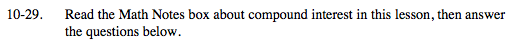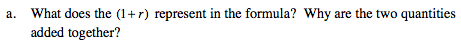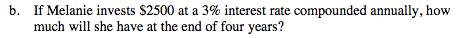### Home > MC2 > Chapter 10 > Lesson 10.1.3 > Problem10-29

10-29.Multiplying the principal amount by 1 results in the original amount. Multiplying the principal by the rate results in the amount gained from interest.

These values are added to calculate the percent based on the previous balance, not just the principal.$\text{Use}\; {\it A} = {\it P}(1 + {\it r})^{\it n}.$

Where P is principal, r is the interest rate, and n is time.

$(2500)(1+0.03)^{4}$

A = \$2813.77